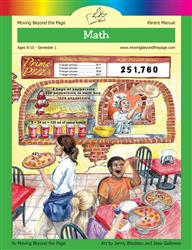Age 5-7 Reading Is Now Available!

# Common Core Alignment

CCSS.Math.Content.4.OA.1 - Interpret a multiplication equation as a comparison, e.g., interpret 35 = 5 × 7 as a statement that 35 is 5 times as many as 7 and 7 times as many as 5. Represent verbal statements of multiplicative comparisons as multiplication equations.

## 5: MathUnit 2: The Four Operations
Lesson 3: Multiplication and Its Properties